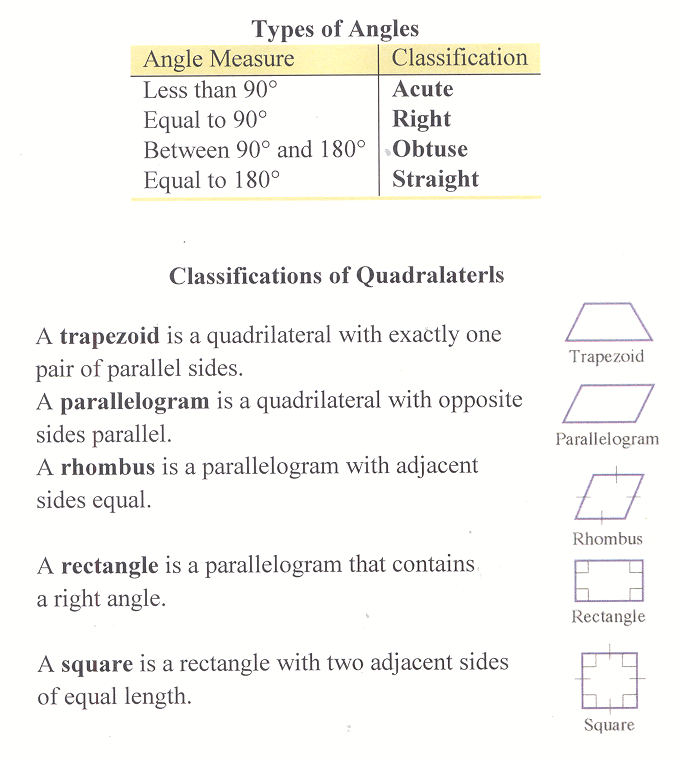# Section 7.2: Polygons and Angles

## 7.2 Outline

1. Angles
1. vertex (pl. vertices)
2. angle
3. notation for angles
4. equal angles
5. degree
6. classifications
1. acute
2. right
3. obtuse
4. straight
7. congruent angles
8. complementary angles
9. supplementary angles
11. vertical angles
2. Polygons
1. definition
2. regular polygon
3. classifications
1. classification
2. trapezoid
3. parallelogram
4. rhombus
5. rectangle
6. square
3. Angles with parallel line.
1. transversal
2. vertical angles are congruent
3. alternate interior angles
4. alternate exterior angles
5. corresponding angles
4. Perpendicular lines
1. definition
2. horizontal line
3. vertical line

## 7.2 Essential IdeasVertical angles are congruent.

Alternate interior angles are pairs of angles whose interiors lie between the parallel lines, but on opposite sides of the transversal. Alternate interior angles are congruent.

Alternate exterior angles are pairs of angles that lie outside the parallel lines, but on opposite sides of the transversal. Alternate exterior angles are congruent.

Corresponding angles are two nonadjacent angles whose interiors lie on the same side of the transversal such that one angle lies between the parallel lines and the other does not. Corresponding angles are congruent.